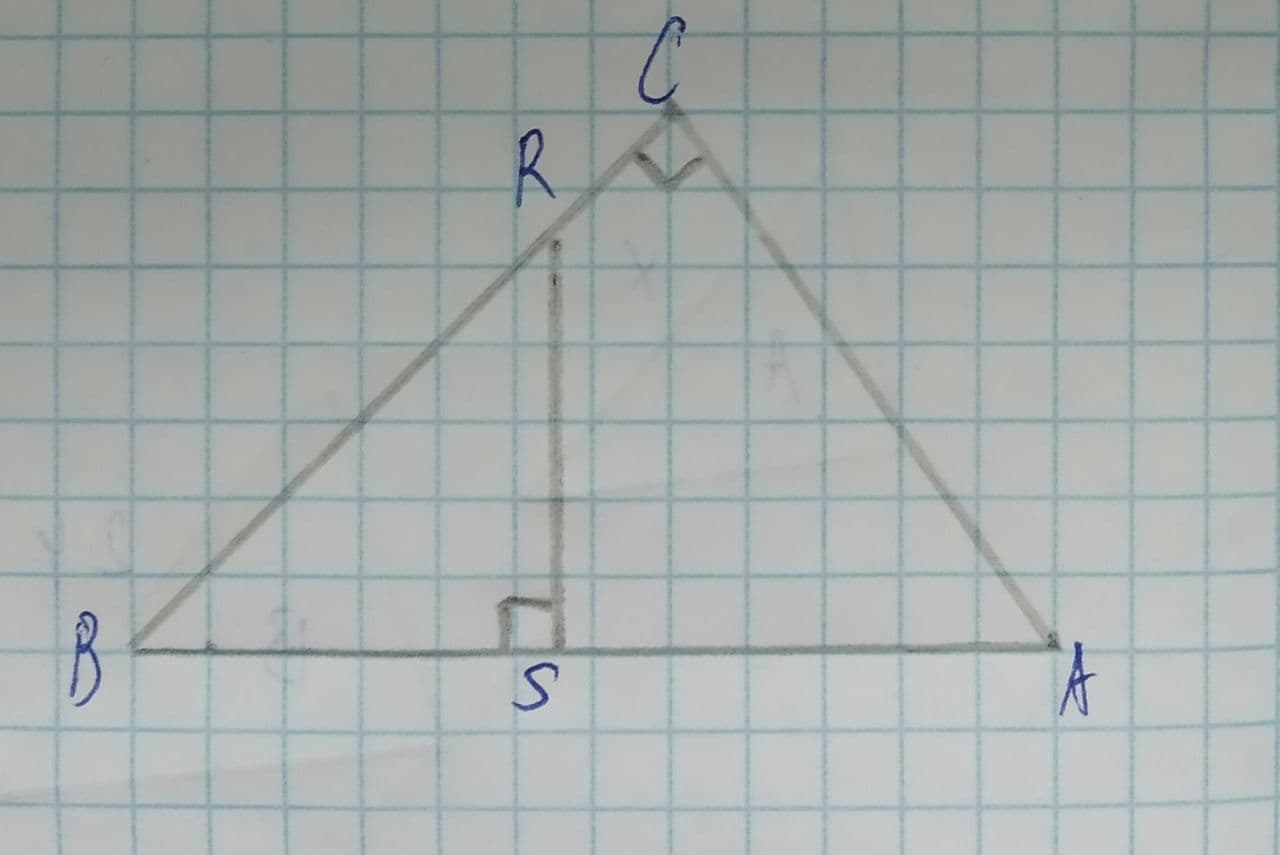Question# Given \overline{RS}\perp\overline{AB}, \overline{CB}\perp\overline{AC}. Prove \triangle BSR\sim \triangle BCA

Similarity
ANSWEREDGiven: $$\displaystyle\overline{{{R}{S}}}\perp\overline{{{A}{B}}},\overline{{{C}{B}}}\perp\overline{{{A}{C}}}$$
Prove: $$\displaystyle\triangle{B}{S}{R}\sim\triangle{B}{C}{A}$$2021-08-15

Now,
$$\displaystyle\overline{{{R}{S}}}\perp\overline{{{A}{B}}}\Rightarrow{m}\angle{R}{S}{B}={90}^{{\circ}}$$
And
$$\displaystyle\overline{{{C}{B}}}\perp\overline{{{A}{C}}}\Rightarrow{m}\angle{A}{C}{B}={90}^{{\circ}}$$
To prove: $$\displaystyle\triangle{B}{S}{R}\sim\triangle{B}{C}{A}$$
In $$\displaystyle\triangle{B}{S}{R}\ {\quad\text{and}\quad}\ \triangle{B}{C}{A}$$
$$\displaystyle{m}\angle{R}{S}{B}={m}\angle{A}{C}{B}={90}^{{\circ}}$$
$$\displaystyle{m}\angle{R}{B}{S}={m}\angle{A}{B}{C}$$ = common angle
$$\displaystyle\therefore\triangle{B}{S}{R}\sim\triangle{B}{C}{A}$$ by Angle−Angle similarity theorem
(Angle−Angle similarity theorem:If two angles of one triangle are equal to two angles of another triangle,then the triangles are similar)
Hence, proved.